Related Articles
• Last Updated : 25 Jun, 2019

Prerequisite: Facebook API | Set-1, Set-2

1. search
2. get_connections
3. get_allconnections

## `search `method:

The valid value types are place and placetopic.

Parameters:
id: It is a string containing a valid value.
args: This is optional and they need to passed a query params.

Example: Get details of all places that are near Connaught Place Delhi. The Latitude and Longitude of Connaught Place are – 28.6304, 77.2177

 `import` `json ` `import` `facebook ` ` `  `def` `main(): ` `    ``token ``=` `"Please replace with your access token"` `    ``graph ``=` `facebook.GraphAPI(token) ` ` `  `    ``places ``=` `graph.search(``type` `=``'place'``, center ``=``'28.6304, 77.2177'``,  ` `                                            ``fields ``=``'name, location'``) ` ` `  `    ``for` `place ``in` `places[``'data'``]: ` `        ``print``(``'%s %s'` `%``(place[``'name'``].encode(), place[``'location'``].get(``'zip'``))) ` `     `  `if` `__name__ ``=``=` `'__main__'``: ` `    ``main() `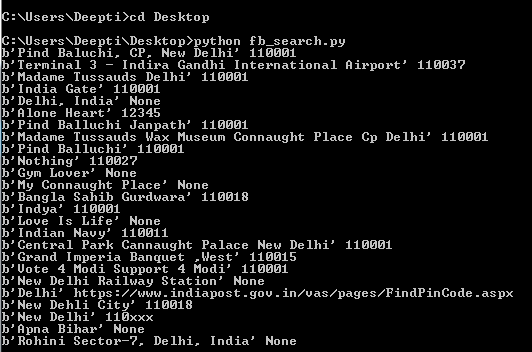Please refer to the link Search Reference for complete list of fields that can used.

## `get_connections `method :

This methods aims at returning all connections or we can say Edges for the mentioned object as dict.

Parameters:
id:A string specifying the unique id for the resource under question.
connection name: A string specifying the connection or edges between the objects.

If connection name parameter is left empty then `get_connections `method will simply return the basic information of the authenticated user.

Example #1: We want to find out the total count of the number of Friends of the active user.

 `import` `json ` `import` `facebook ` ` `  `def` `main(): ` `    ``token ``=` `"Please replace this with your access token"` `    ``graph ``=` `facebook.GraphAPI(token) ` `    ``friends ``=` `graph.get_connections(``id` `=``'me'``, connection_name ``=``'friends'``) ` `    ``print``(json.dumps(friends, indent ``=` `4``)) ` ` `  `if` `__name__ ``=``=` `'__main__'``: ` `    ``main() `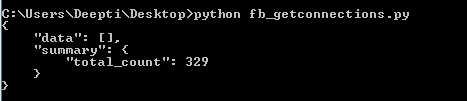Example #2: We want to get list of all posts from a page. For this, we will be using get_connections and later on, we will demonstrate how to use `get_all_connections `method.

 `import` `json ` `import` `facebook ` ` `  `def` `main(): ` `    ``token ``=` `"Please replace this with your PAGE Access Token"` `    ``graph ``=` `facebook.GraphAPI(token) ` `    ``posts_25 ``=` `graph.get_connections(``id` `=``'PAGE_ID'``, connection_name ``=``'posts'``, ` `                                                  ``fields ``=``'id, created_time'``) ` ` `  `    ``print``(json.dumps(posts_25, indent ``=` `4``)) ` ` `  `if` `__name__ ``=``=` `'__main__'``: ` `    ``main()  `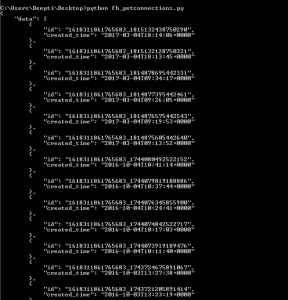Note: This example by default print latest 25 posts. You can add a filter for the post using limit(…) to set the limit for the number of the posts.

Example #3: In this example we will use get_connections method to print all the comments for the posts in `reverse_chronological `order, show sub comments including hidden comments and also show the total count of the comments.

 `import` `json ` `import` `facebook ` ` `  `def` `main(): ` `    ``token ``=` `"Please replace this with your PAGE Access Token"` `    ``graph ``=` `facebook.GraphAPI(token) ` `    ``posts_25 ``=` `graph.get_connections(``id` `=``'POST_ID'``, connection_name ``=``'comments'``,  ` `                                     ``include_hidden ``=` `True``, order ``=``'reverse_chronological'``, ` `                                     ``filter` `=``'stream'``, summary ``=``'total_count'``) ` ` `  `    ``print``(json.dumps(posts_25, indent ``=` `4``)) ` ` `  `if` `__name__ ``=``=` `'__main__'``: ` `    ``main()  `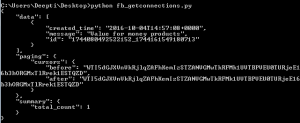## `get_all_connections `method :

Iterates over all pages returned by a get_connections call and yields the individual items.

Parameters:
id:A string specifying the unique id for the resource under question.
connection name: A string specifying the connection or edges between the objects.

Example #1: In this example I have used get_all_connections to list all the posts from a Page listed using the since datetime parameter.

 `import` `json ` `import` `facebook ` `from` `datetime ``import` `datetime ` `  `  `def` `main(): ` `    ``token ``=` `"Please replace this with your PAGE Access Token"` `    ``graph ``=` `facebook.GraphAPI(token) ` `    ``posts_all ``=` `graph.get_all_connections(``id` `=``'PAGE_ID'``, connection_name ``=``'posts'``, ` `                                           ``fields ``=``'created_time, id'``, ` `                                           ``since ``=` `datetime(``2017``, ``1``, ``1``, ``0``, ``0``, ``0``)) ` ` `  `    ``for` `ind, post ``in` `enumerate``(posts_all): ` `     ``print``(json.dumps(ind, indent ``=` `4``)) ` `     ``print``(json.dumps(post, indent ``=` `4``)) ` `     `  `  `  `if` `__name__ ``=``=` `'__main__'``: ` `    ``main() `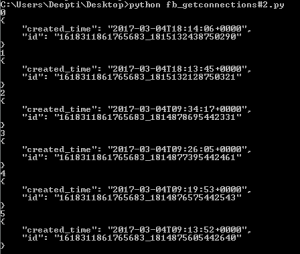References: